Technical Article

# The Basics of Power Semiconductor Devices: Structures, Symbols, and Operations

June 13, 2015 by Editorial Team

## This technical article is dedicated to the review of the following power electronics devices which act as solid-state switches in the circuits. These act as a switch without any mechanical movement.

The Structures, Electronic Symbols, Basic Operations and Several Characteristics Representations of Power Semiconductor Devices

Beginner

### Power Electronic Devices That Act As Solid-State Switches

This technical article is dedicated to the review of the following power electronic devices which act as solid-state switches in the circuits. They act as a switch without any mechanical movement.

• Power Diodes
• Metal-Oxide-Semiconductor Field-Effect Transistor (MOSFET)
• Bipolar -Junction Transistor (BJT)
• Insulated-Gate Bipolar Transistor (IGBT)
• Thyristors (SCR, GTO, MCT)

Solid-state devices are completely made from a solid material and their flow of charges is confined within this solid material. This name “solid state” is often used to show a difference with the earlier technologies of vacuum and gas-discharge tube devices; and  also to exclude the conventional electro-mechanical devices (relays, switches, hard drives and other devices with moving parts).

The transistor by Bell Labs in 1947 was the first solid-state device to come into commercial use later in the 1960s. In this article, similar solid-state devices such as power diode, power transistor, MOSFET, thyristor and its two-transistor model, triac, gate turn-off thyristor (GTO), insulated-gate bipolar transistor (IGBT) and their characteristics (such as i-v characteristics and turn-off characteristics) is also presented. In power electronics circuitry, these switches act in saturation region and work in linear region in the analog circuitry such as in power amplifiers and linear regulators. This makes these switches highly efficient since there are lesser losses during the power processing.

### Power Diodes

A power diode has a P-I-N structure as compared to the signal diode having a P-N structure. Here, I (in P-I-N) stands for intrinsic semiconductor layer to bear the high-level reverse voltage as compared to the signal diode (n- , drift region layer shown in Fig. 2). However, the drawback of this intrinsic layer is that it adds noticeable resistance during forward-biased condition. Thus, power diode requires a proper cooling arrangement for handling large power dissipation.  Power diodes are used in numerous applications including rectifier, voltage clamper, voltage multiplier and etc. Power diode symbol is the same as of the signal diode as shown in Fig.1.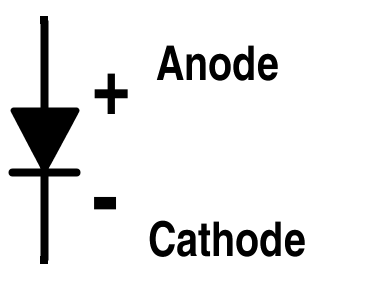Figure 1. Symbol for Power DiodeFigure 2. Structure of Power Diode

Other features that are incorporated in the power diode letting it handle larger power are:

(a) Use of guard rings

(b) Coating of Silicon Dioxide layer

Guard rings are of p-type that prevents their depletion layer merge with the depletion layer of the reverse-biased p-n junction. The guard rings prevent the radius of the curvature of the depletion layer boundary to become too narrow which increases the breakdown strength. Coating of SiO2 layer helps in limiting the electric field at the surface of the power diode.

If thickness of lightly doped I layer (n- layer) > depletion layer width at breakdown ⇒ Non-punch through power diode.

(This means depletion layer has not punched through the lightly-doped n-layer.)

If thickness of I layer < depletion layer width at breakdown ⇒ Punch through power diode.

#### Characteristics of Power Diode

The two types of characteristics of a power diode are shown in Fig. 3 and Fig. 4 named as follows:

(i) Amp-volt characteristics (i-v characteristics)

(ii) Turn-off characteristics (or reverse-recovery characteristics)Figure 3. Amp-Volt Characteristics of Power Diode

Cut-in voltage is the value of the minimum voltage for VA (anode voltage) to make the diode works in forward conducting mode. Cut-in voltage of signal diode is 0.7 V while in power diode it is 1 V. So, its typical forward conduction drop is larger.   Under forward-bias condition, signal diode current increases exponentially and then increases linearly. In the case of the power diode, it almost increases linearly with the applied voltage as all the layers of P-I-N remain saturated with minority carriers under forward bias. Thus, a high value of current produces results in voltage drop which mask the exponential part of the curve. In reverse-bias condition, small leakage current flows due to minority carriers until the avalanche breakdown appears as shown in Fig. 3.Figure 4. Turn-Off Characteristics of Power Diode: a) Variation of Forward Current if ; b) Variation of Forward Voltage Drop vf ; c) Variation of Power Loss

After the forward diode comes to null, the diode continues to conduct in the opposite direction because of the presence of stored charges in the depletion layer and the p or n-layer. The diode current flows for a reverse-recovery time trr. It is the time between the instant forward diode current becomes zero and the instant reverse-recovery current decays to 25 % of its reverse maximum value.

Time T:  Charges stored in the depletion layer removed.

Time Tb :  Charges from the semiconductor layer is removed.

Shaded area in Fig 4.a represents stored charges QR which must be removed during reverse-recovery time trr.

Power loss across diode = v* if    (shown in Fig. 4.c)

As shown, major power loss in the diode occurs during the period tb.

Recovery can be abrupt or smooth as shown in Fig. 5. To know it quantitatively, we can use the S – factor.

Ratio Tb/T: Softness factor or S-factor.

S-factor: measure of the voltage transient that occurs during the time the diode recovers.

S-factor = 1 ⇒ low oscillatory reverse-recovery process. (Soft –recovery diode)

S-factor <1  ⇒ large oscillatory over voltage (snappy-recovery diode or fast-recovery diode).

Power diodes now exist with forward current rating of 1A to several thousand amperes with reverse-recovery voltage ratings of 50V to 5000V or more.Figure 5. Reverse-Recovery Characteristics for Power Diode

Schottky Diode: It has an aluminum-silicon junction where the silicon is an n-type. As the metal has no holes, there is no stored charge and no reverse-recovery time. Therefore, there is only the movement of the majority carriers (electrons) and the turn-off delay caused by recombination process is avoided. It can also switch off much faster than a p-n junction diode. As compared to the p-n junction diode it has:

(a) Lower cut-in voltage

(b) Higher reverse leakage current

(c) Higher operating frequency

Application: high-frequency instrumentation and switching power supplies.Figure 6. Schottky Diode Symbol and Current-Voltage Characteristics Curve

### Metal-Oxide Semiconductor Field-Effect Transistor (MOSFET)

MOSFET is a voltage-controlled majority carrier (or unipolar) three-terminal device. Its symbols are shown in Fig. 7 and Fig. 8. As compared to the simple lateral channel MOSFET for low-power signals, power MOSFET has different structure. It has a vertical channel structure where the source and the drain are on the opposite side of the silicon wafer as shown in Fig. 10. This opposite placement of the source and the drain increases the capability of the power MOSFET to handle larger power.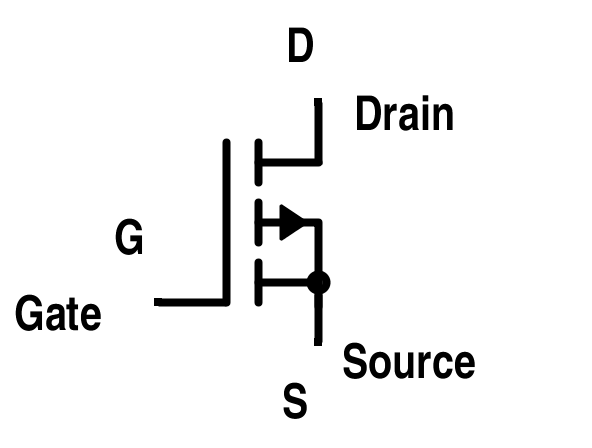Figure 7. MOSFET Symbol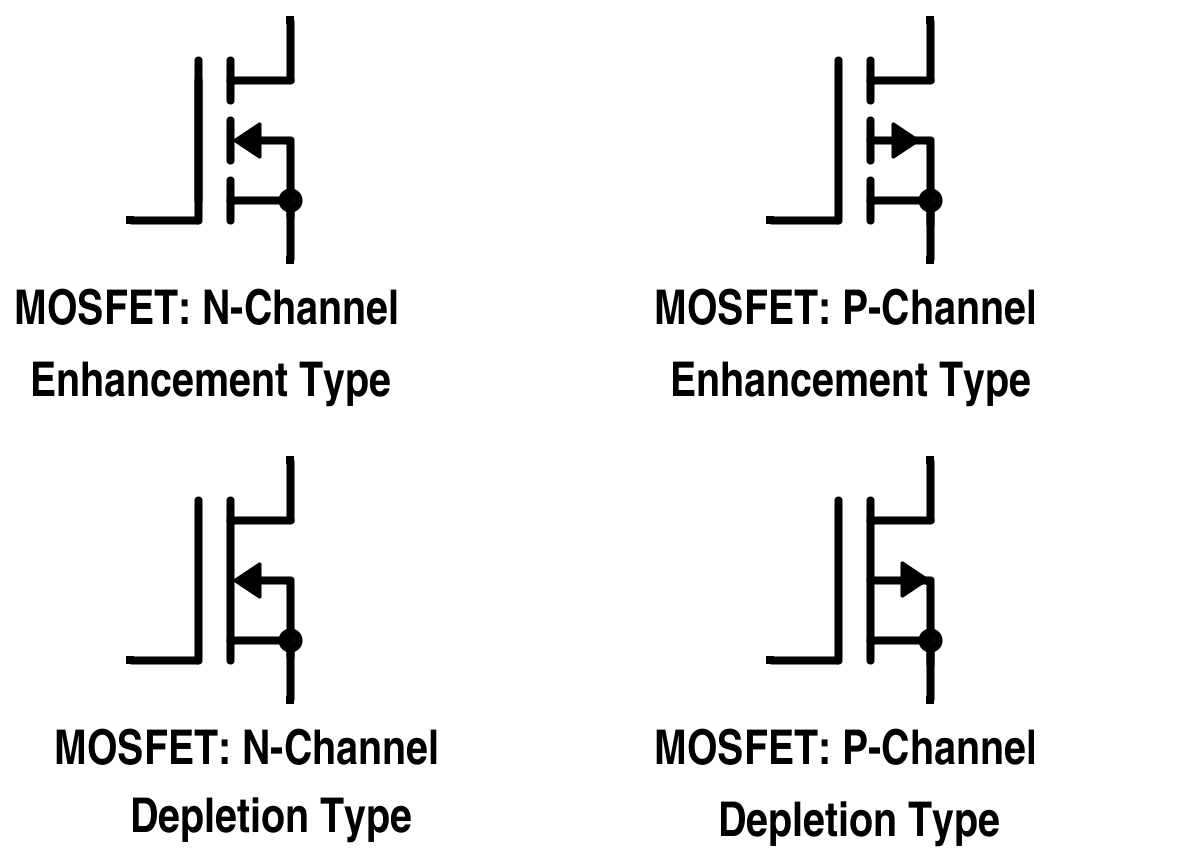Figure 8. MOSFET Symbols for Different Modes

In all of these connections, substrates are internally connected. But in cases where it is connected externally, the symbol will change as shown in the n-channel enhancement type MOSFET in Fig. 9. N-channel enhancement type MOSFET is more common due to high mobility of electrons.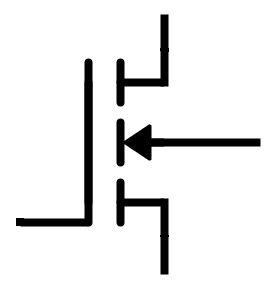Figure 9. N-channel Enhancement-Type MOSFET with Substrate Connected ExternallyFigure 10. Cross-Sectional View of the Power MOSFET

Basic circuit diagram and output characteristics of an n-channel enhancement power MOSFET with load connected are in Fig. 11 and Fig. 12 respectively.Figure 11. Power MOSFET Structural View with Connections

Drift region shown in Fig. 11 determines the voltage-blocking capability of the MOSFET.

When VGS = 0,

⇒ VDD makes it reverse biased and no current flows from drain to source.

When VGS > 0,

⇒ Electrons form the current path as shown in Fig. 11. Thus, current from the drain to the source flows. Now, if we will increase the gate-to-source voltage, drain current will also increase.Figure 12. Drain Current (ID) vs Drain-to-Source Voltage (VDS) Characteristics Curves

For lower value of VDS, MOSFET works in a linear region where it has a constant resistance equal to VDS / ID. For a fixed value of VGS and greater than threshold voltage VTH, MOSFET enters a saturation region where the value of the drain current has a fixed value.Figure 13. Output Characteristics with Load Line

If XY represents the load line, then the X-point represents the turn-off point and Y-point is the turn-on point where VDS = 0 (as voltage across the closed switch is zero). The direction of turning on and turning off process is also shown in Fig. 13.

Besides the output characteristics curves, transfer characteristics of power MOSFET is also shown in Fig. 14.Figure 14. Gate-to-Source Voltage vs. Drain Current Characteristics for Power MOSFET

Here, VTH is the minimum positive voltage between gate and the source above which MOSFET comes in on-state from the off-state. This is called threshold voltage. It is also shown in the output characteristics curve in Fig. 12.

Close view of the structural diagram given in Fig. 11 reveals that there exists a fictitious BJT and a fictitious diode structure embedded in the power MOSFET as shown in Fig. 15.

As source is connected to both base and emitter of this parasitic BJT, emitter and base of the  BJT are short circuited. That means this BJT acts in cut-off state.Figure 15. Fictitious BJT and Fictitious Diode in the Power MOSFET

Fictitious diode anode is connected to the source and its cathode is connected to the drain. So, if we apply the negative voltage VDD across the drain and source, it will be forward biased. That means, the reverse-blocking capability of the MOSFET breaks. Thus, this can be used in inverter circuit for reactive loads without the need of excessive diode across a switch. Symbolically, it is represented in Fig. 16.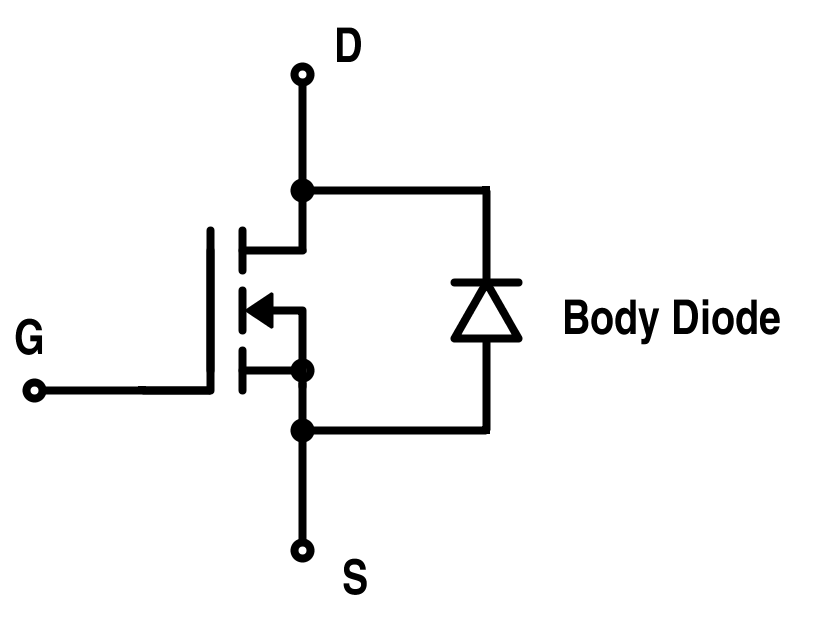Figure 16. MOSFET Representation with Internal Body Diode

Although MOSFET internal body diode has sufficient current and switching speed for most of the applications, there may be some applications where the use of ultra-fast diodes is required. In such cases, an external fast-recovery diode is connected in an antiparallel manner. But a slow-recovery diode is also required to block the body diode action as given in Fig. 17.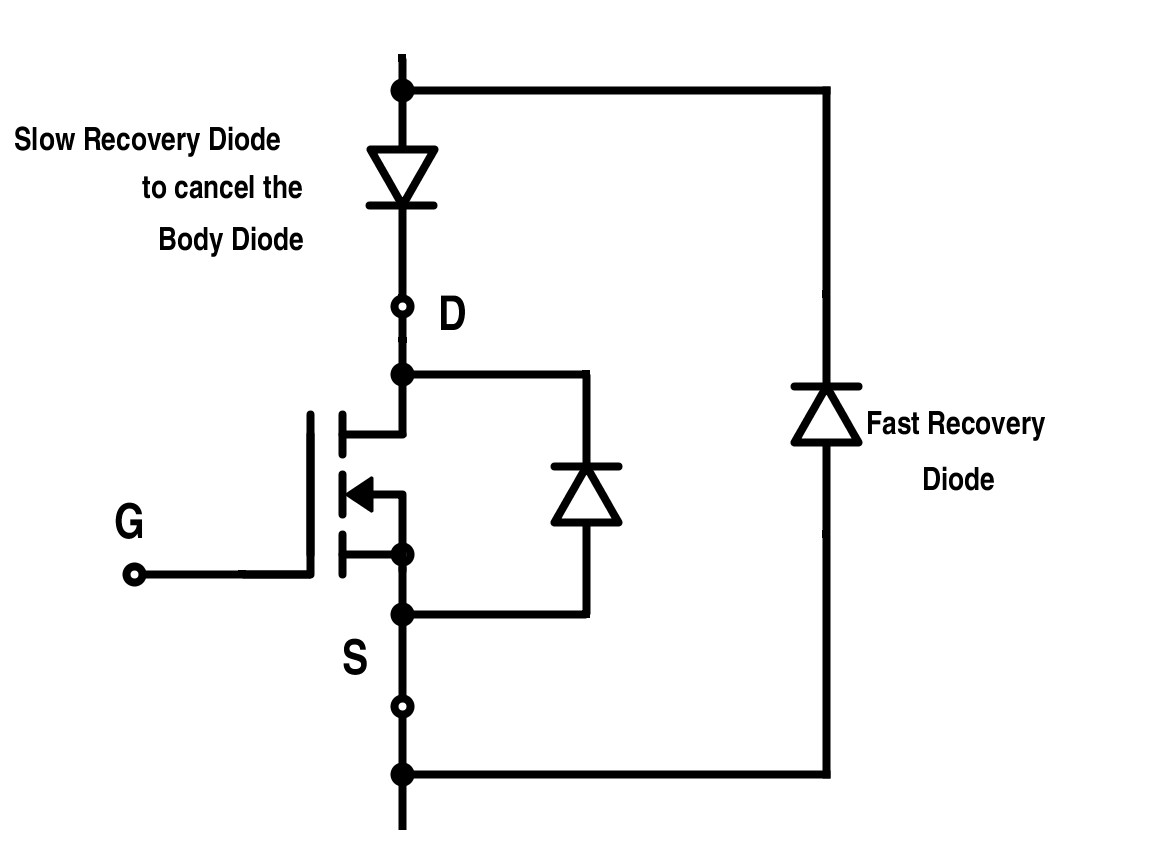Figure 17. Implementation of Fast-Recovery Diode for Power MOSFET

One of the important parameters that affects the switching characteristics is the body capacitances existing between its three terminals i.e. drain, source and gate. Its representation is shown in Fig. 18.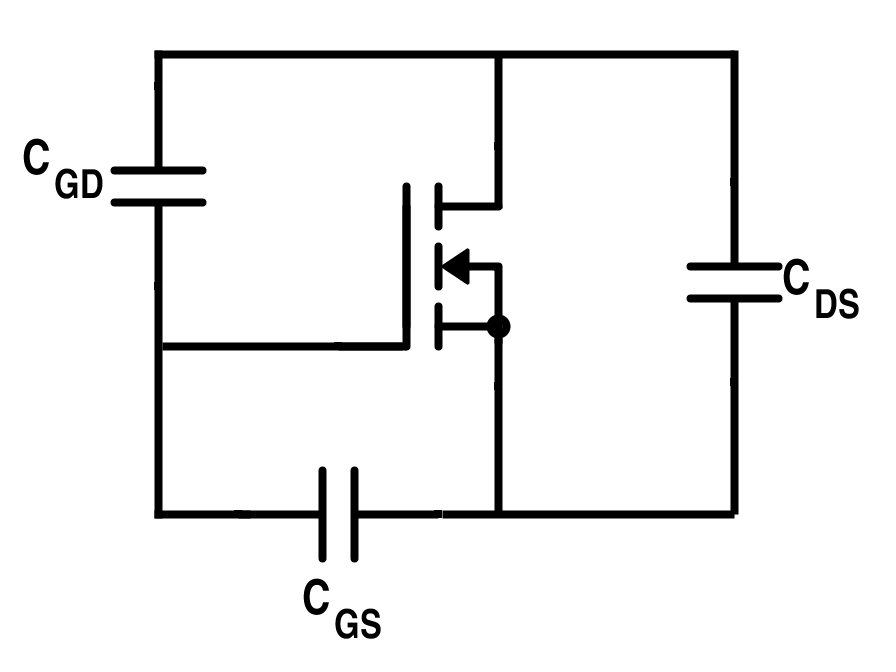Figure 18. MOSFET Representation Showing Junction Capacitances

Parameters CGS, CGD and CDS are all non-linear in nature and given in the device’s data sheet of a particular MOSFET. They also depend on the DC bias voltage and the device’s structure or geometry. They must be charged through gate during turn-on process to actually turn on the MOSFET. The drive must be capable of charging and discharging these capacitances to switch on or switch off the MOSFET.

Thus, the switching characteristics of a power MOSFET depend on these internal capacitances and the internal impedance of the gate drive circuits. Also, it depends on the delay due to the carrier transport through the drift region. Switching characteristics of power MOSFET are shown in Fig. 19 and Fig. 20.Figure 19. Turn-on Characteristics of Power MOSFET

There is a delay from t0 to t1 due to charging of input capacitance up to its threshold voltage VTH. Drain current in this duration remains at zero value. This is called a delay time. There is a further delay from t1 to t2 during which the gate voltage rises to VGS, a voltage required to drive the MOSFET into on-state.  This is called the rise time. This total delay can be reduced by using a low-impedance drive circuit. The gate current during this duration decreases exponentially as shown. For the time greater than t2, the drain current ID has reached its maximum constant value I. As drain current has reached the constant value, the gate-to-source voltage is also constant as shown in the transfer characteristics of MOSFET in Fig. 20.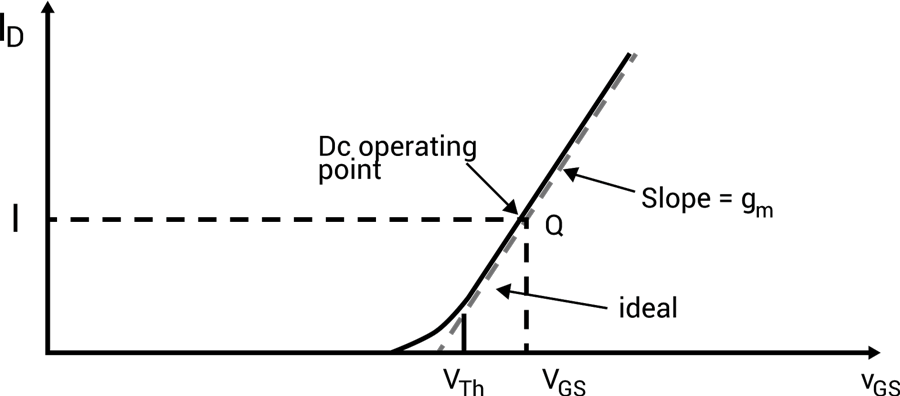Figure 20. Transfer Characteristics of Power MOSFET with Operating Point

For turn-off characteristics, assume that the MOSFET is already in the switched-on situation with steady state. As t = t0, gate voltage is reduced to zero value; CGS and CGD start to discharge through gate resistance RG. This causes a turn-off delay time up to t1 from t0 as shown in Fig. 21. Assuming the drain-to-source voltage remains fixed. During this duration, both VGS and IG decreases in magnitude, drain current remains at a fixed value drawing current from CGD and CGS.Figure 21. Turn-Off Characteristics of Power MOSFET

For the time where t> t > t1, gate-to-source voltage is constant. Thus, the entire current is now being drawn from CGD. Up to time t3, the drain current will almost reach zero value; which turns off the MOSFET. This time is known as the fall time, this is when the input capacitance discharges up to the threshold value. Beyond t3, gate voltage decreases exponentially to zero until the gate current becomes zero.

### Power Bipolar Junction Transistor (BJT)

Power BJT is used traditionally for many applications. However, IGBT (Insulated-Gate Bipolar Transistor) and MOSFET (Metal-Oxide-Semiconductor Field-Effect Transistor) have replaced it for most of the applications but still they are used in some areas due to its lower saturation voltage over the operating temperature range. IGBT and MOSFET have higher input capacitance as compared to BJT. Thus, in case of IGBT and MOSFET, drive circuit must be capable to charge and discharge the internal capacitances.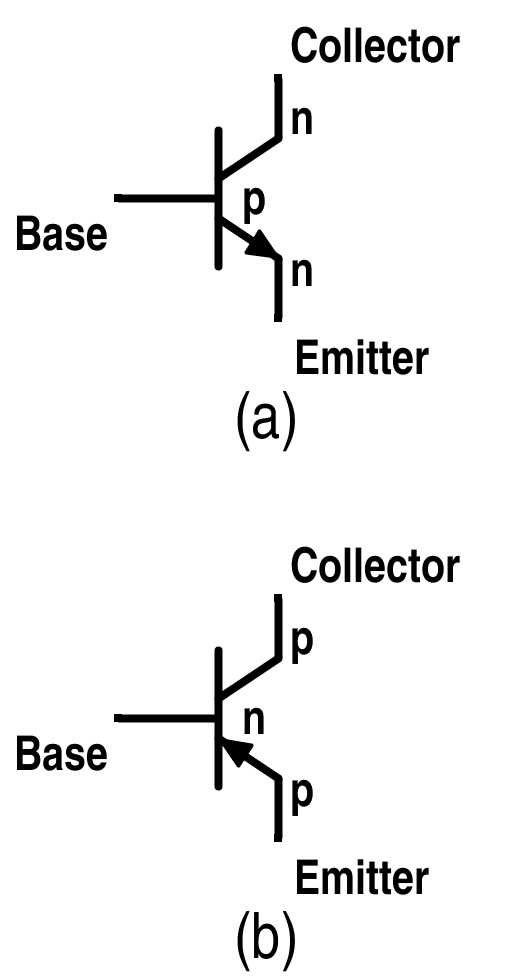Figure 22. (a) NPN BJT (b) PNP BJT

The BJT is a three-layer and two-junction npn or pnp semiconductor device as given in Fig. 22. (a) and (b).

Although BJTs have lower input capacitance as compared to MOSFET or IGBT, BJTs are considerably slower in response due to low input impedance. BJTs use more silicon for the same drive performance.

In the case of MOSFET studied earlier, power BJT is different in configuration as compared to simple planar BJT. In planar BJT, collector and emitter is on the same side of the wafer while in power BJT it is on the opposite edges as shown in Fig. 23. This is done to increase the power-handling capability of BJT.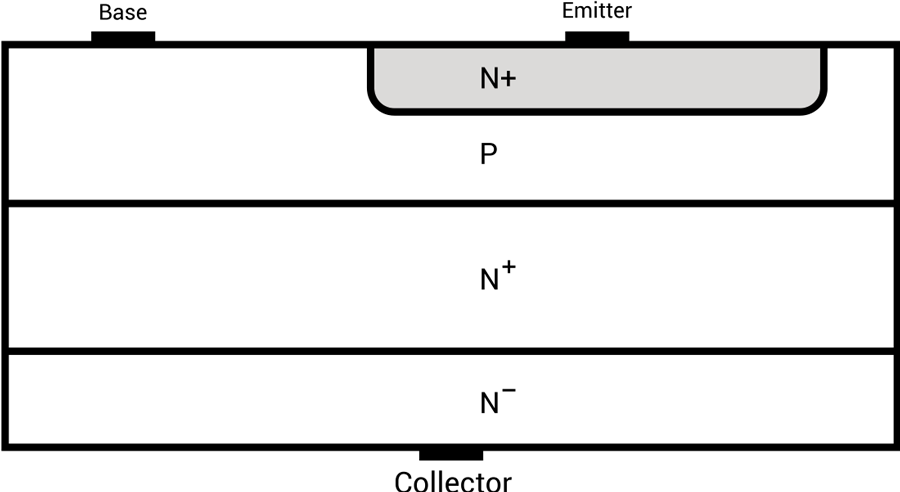Figure 23. Power BJT PNP Structure

Power n-p-n transistors are widely used in high-voltage and high-current applications which will be discussed later.

Input and output characteristics of planar BJT for common-emitter configuration are shown in Fig. 24. These are current-voltage characteristics curves.Figure 24. Input Characteristics and Output Characteristics for the Common-Emitter Configuration of Planar BJT respectively

Characteristic curves for power BJT is just the same except for the little difference in its saturation region. It has additional region of operation known as quasi-saturation as shown in Fig. 25.Figure 25. Power BJT Output Characteristics Curve

This region appears due to the insertion of lightly-doped collector drift region where the collector base junction has a low reverse bias. The resistivity of this drift region is dependent on the value of the base current.  In the quasi-saturation region, the value of ß decreases significantly. This is due to the increased value of the collector current with increased temperature. But the base current still has the control over the collector current due to the resistance offered by the drift region.  If the transistor enters in hard saturation region, base current has no control over the collector current due to the absence of the drift region and mainly depends on the load and the value of VCC.

A forward-biased p-n junction has two capacitances named depletion layer capacitance and diffused capacitance. While a reverse bias junction has only a depletion capacitance in action. Value of these capacitances depends on the junction voltage and construction of the transistor. These capacitances come into role during the transient operation i.e. switching operations. Due to these capacitances, transistor does not turn on or turn off instantly.

Switching characteristics of power BJT is shown in Fig.26.  As the positive base voltage is applied, base current starts to flow but there is no collector current for some time. This time is known as the delay time (td) required to charge the junction capacitance of the base to emitter to 0.7 V approx. (known as forward-bias voltage). For t > td, collector current starts rising and VCE starts to drop with the magnitude of 9/10th of its peak value. This time is called rise time, required to turn on the transistor. The transistor remains on so long as the collector current is at least of this value.

For turning off the BJT, polarity of the base voltage is reversed and thus the base current polarity will also be changed as shown in Fig. 26. The base current required during the steady-state operation is more than that required to saturate the transistor. Thus, excess minority carrier charges are stored in the base region which needs to be removed during the turn-off process. The time required to nullify this charge is the storage time, ts. Collector current remains at the same value for this time. After this, collector current starts decreasing and base-to-emitter junction charges to the negative polarity; base current also get reduced.Figure 26. Turn-On and Turn-Off Characteristics of BJT

### Insulated-Gate Bipolar Transistor (IGBT)

IGBT combines the physics of both BJT and power MOSFET to gain the advantages of both worlds. It is controlled by the gate voltage. It has the high input impedance like a power MOSFET and has low on-state power loss as in case of BJT.  There is no even secondary breakdown and not have long switching time as in case of BJT. It has better conduction characteristics as compared to MOSFET due to bipolar nature. It has no body diode as in case of MOSFET but this can be seen as an advantage to use external fast recovery diode for specific applications. They are replacing the MOSFET for most of the high voltage applications with less conduction losses. Its physical cross-sectional structural diagram and equivalent circuit diagram is presented in Fig. 27 to Fig. 29. It has three terminals called collector, emitter and gate.Figure 27. IGBT Structure View

There is a p+ substrate which is not present in the MOSFET and responsible for the minority carrier injection into the n-region. Gain of NPN terminal is reduced due to wide epitaxial base and n+ buffer layer.

There are two structures of IGBTs based on doping of buffer layer:

a) Punch-through IGBT: Heavily doped n buffer layer ➔ less switching time

b) Non-Punch-through IGBT: Lightly doped n buffer layer ➔ greater carrier lifetime ➔ increased conductivity of drift region ➔ reduced on-state voltage drop

(Note: ➔ means implies)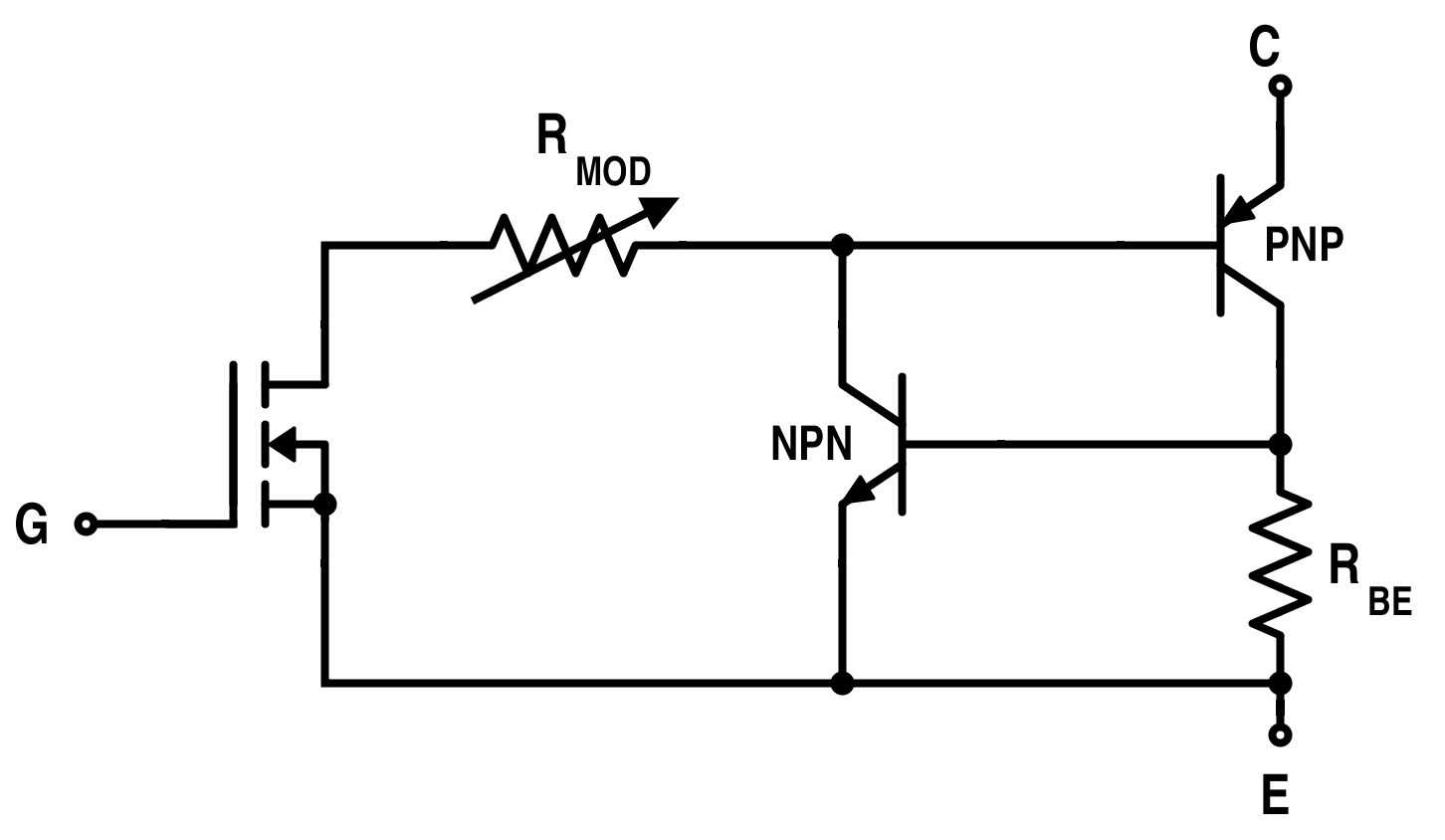Figure 28. Equivalent Circuit for IGBT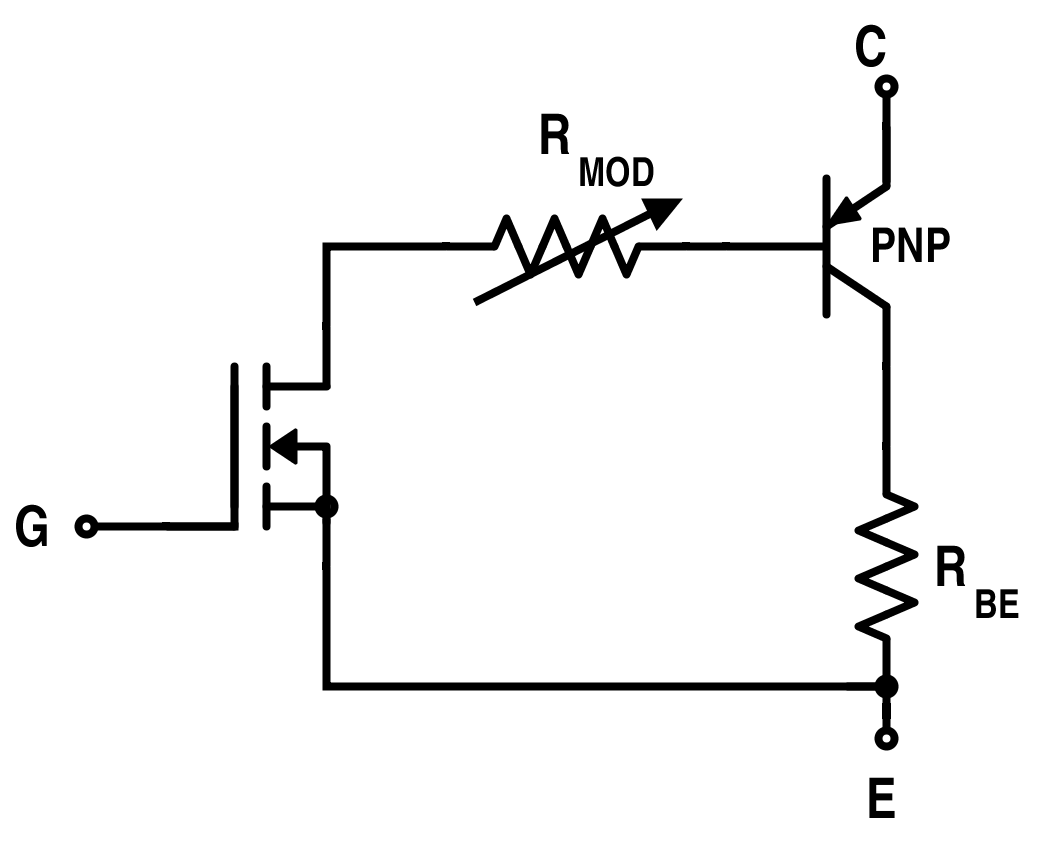Figure 29. Simplified Equivalent Circuit for IGBT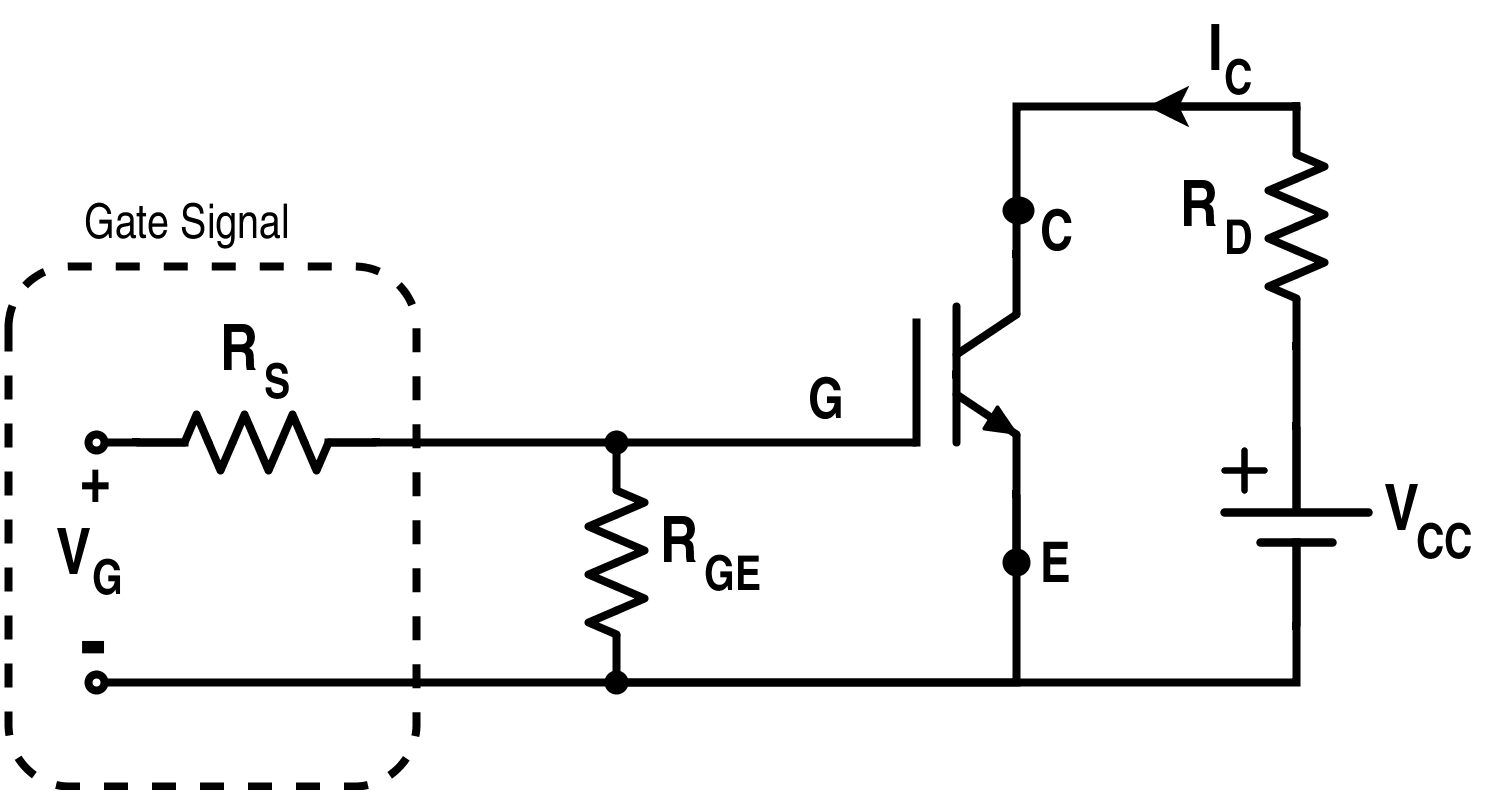Figure 30. Circuit Diagram for IGBT

Based on this circuit diagram given in Fig.30, forward characteristics and transfer characteristics are obtained which are given in Fig.31 and Fig.32. Its switching characteristic is also shown in Fig. 33.Figure 31. Forward Characteristics for IGBTFigure 32. Transfer Characteristics of IGBTFigure 33. Turn-On and Turn-Off Characteristics of IGBT

(Note: Tdn : delay time ; Tr: rise time ; Tdf : delay time ; Tf1: initial fall time ; Tf2: final fall time)

### Thyristors (SCR, GTO, MCT)

Thyristors are the family of solid-state devices extensively used in power electronics circuitry such as SCR (silicon-controlled rectifier), DIAC (diode on AC), TRIAC (triode on AC), GTO (gate turn-off thyristors), MCT (MOS-controlled thyristor), RCT, PUT, UJT, LASCR, LASCS, SIT, SITh, SIS, SBS, SUS, SBS and etc. SCR is the oldest member and the head of this family; and usually referred with the name “thyristor”.

They are operated as bistable switches that are either working in non-conducting or conducting state. Traditional thyristors are designed without gate-controlled turn-off capability in which the thyristor can come from conducting state to non-conducting state when only anode current falls below the holding current. While GTO is a type of thyristor that has a gate-controlled turn-off capability.

#### SCR

SCR usually has three terminals and four layers of alternating p and n-type materials as shown in Fig. 34. The structure of the thyristor can be split into two sections: npn and pnp transistors for simple analysis purposes as shown in Fig. 36. It has three terminals named as cathode, anode and gate.Figure 34. Structural View of Thyristor

N-base is a high-resistivity region and its thickness is directly dependent on the forward blocking rating of the thyristor. But more width of the n-base indicates a slow response time for switching. Symbol of thyristor is given in Fig. 35.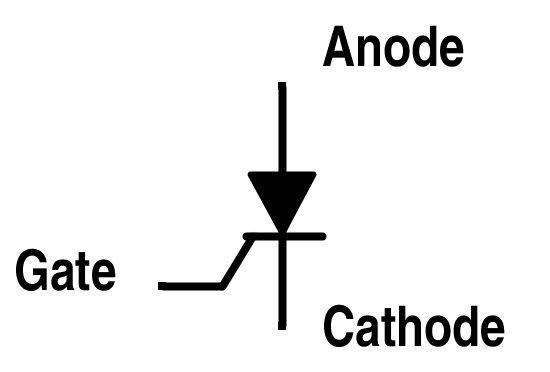Figure 35. Schematic Symbol of ThyristorFigure 36. Two-Transistor Model of a Thyristor (A-Anode, G-Gate and K-Cathode)

Planar construction is used for low-power SCRs. In this type of construction, all the junctions are diffused. For high power, mesa construction is used where the inner layer is diffused and the two outer layers are alloyed on it.

The static characteristic obtained from the circuit given in Fig. 37 is drawn in Fig. 38. It works under three modes: forward conducting mode, forward blocking mode and reverse blocking mode.

The minimum anode current that causes the device to stay at forward conduction mode as it switch from forward blocking mode is called the latching current. If the SCR is already conducting and the anode current is reduced from forward conducting mode to forward blocking mode, the minimum value of anode current to remain at the forward conducting mode is known as the holding current.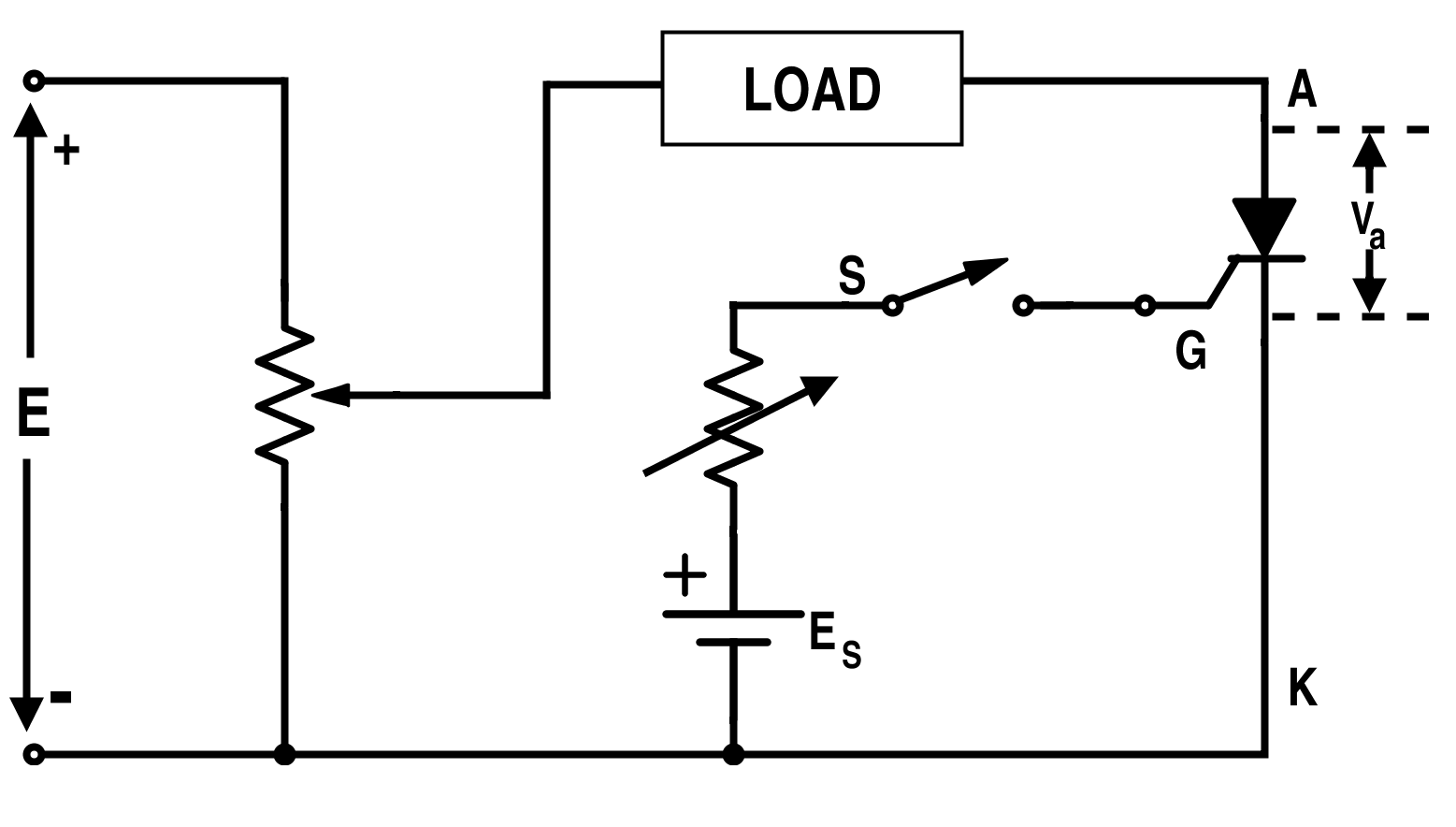Figure 37. Basic Circuit for Getting Voltage and Current Characteristics of ThyristorFigure 38. Static Characteristics Curve of SCR

Switching characteristics of SCR are shown in Fig. 39. Note that it can’t be turned off with the gate. This is due to positive feedback or a regenerative feedback effect.Figure 39. Turn-On and Turn-Off Characteristics of SCR

#### GTO (Gate Turn-off Thyristor)

GTO can be turned on with the positive gate current pulse and turned off with the negative gate current pulse. Its capability to turn off is due to the diversion of PNP collector current by the gate and thus breaking the regenerative feedback effect.

Actually the design of GTO is made in such a way that the pnp current gain of GTO is reduced. Highly doped n spots in the anode p layer form a shorted emitter effect and ultimately decreases the current gain of GTO for lower current regeneration and also the reverse voltage blocking capability. This reduction in reverse blocking capability can be improved by diffusing gold but this reduces the carrier lifetime. Moreover, it requires a special protection as shown in Fig. 43.

Fig. 40 shows the four Si layers and the three junctions of GTO and Fig. 41 shows its practical form. The symbol for GTO is shown in Fig.42.Figure 40. Four Layers and Three Junctions of GTOFigure 41. Practical Form of GTOFigure 42. Symbol of GTO

Overall switching speed of GTO is faster than thyristor (SCR) but voltage drop of GTO is larger. The power range of GTO is better than BJT, IGBT or SCR.

The static voltage current characteristics of GTO are similar to SCR except that the latching current of GTO is larger (about 2 A) as compared to SCR (around 100-500 mA).

The gate drive circuitry with switching characteristics is given in Fig. 43 and Fig. 44.Figure 43. Gate Drive Circuit for GTOFigure 44. Turn-On and Turn-Off Characteristics of GTO

#### MCT (MOS-Controlled Thyristor)

IGBT is an improvement over a BJT using a MOSFET to switch on or switch off the anode current. Similarly, MCT is an improvement over a thyristor with a pair of MOSFETs to switch the current. There are several devices in the MCT’s family but the p-channel is commonly discussed. Its schematic diagram and equivalent circuit is given in Fig. 45 and Fig. 46. Its symbol is given in Fig. 47.Figure 45. Schematic Diagram of P-Type MCT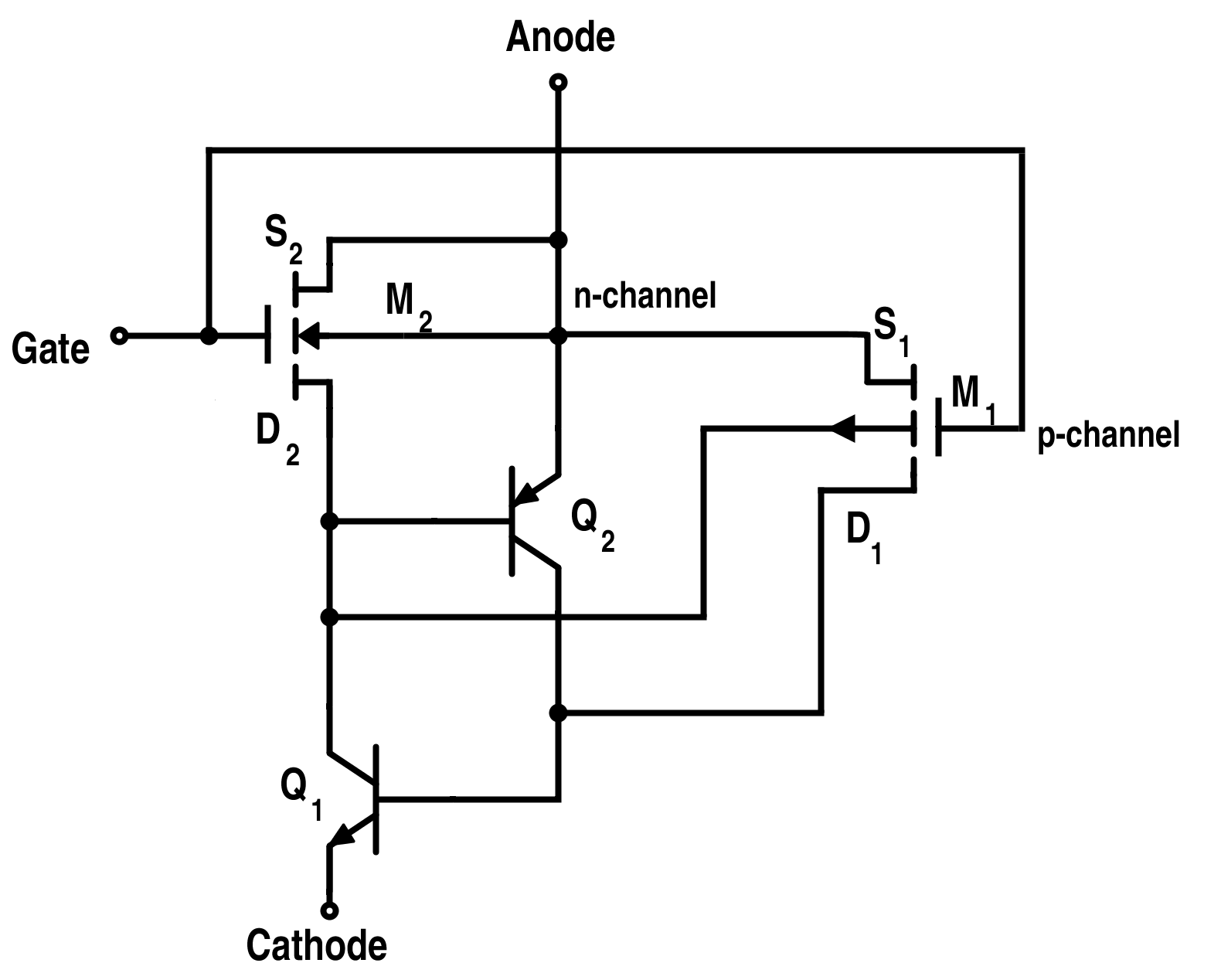Figure 46. Equivalent Circuit for P-Type MCT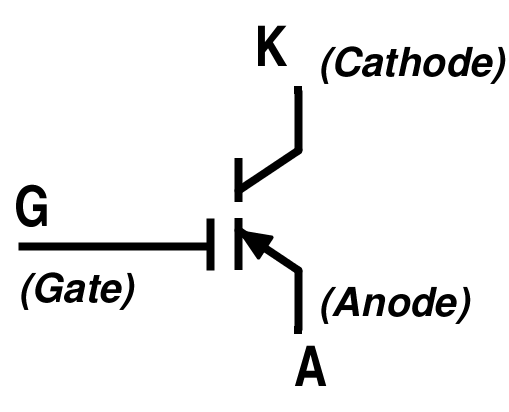Figure 47. Symbol of P-Type MCT

Due to NPNP structure instead of PNPN, anode acts as a reference for gate. NPNP structure is represented by NPN transistor Q1 and a PNP transistor Q2 in the equivalent circuit.

The power required to switch it on or off is small with low switching losses due to its distributed structure across the entire surface of the device. Delay time due to charge storage is also small. It also has a low on-state voltage drop.

When a p-type MCT is in the forward-blocking state, it can be switched on by applying a negative pulse to its gate (with respect to anode). While when an n-channel MCT is in the forward-blocking mode, it can be switched on with the positive gate pulse (with respect to cathode). It will remain on until the device current is reversed or a turn-off pulse is applied to the gate i.e. applying a positive gate pulse for p-type MCT with respect to anode.

This device can bear a high current and high $$\frac{di}{dt}$$ capability. But just like any other devices, it needs to be protected against transient voltages and current spikes with the help of suitable snubbers.  It is used in capacitor discharge applications, circuit breakers, AC-AC or AC-DC conversion. It is an ideal replacement for GTO as it requires a much simpler gate drive and certainly more efficient.

• Share• R
reylehk October 09, 2015

what is the “hole” with a transistor

Like.
•jssamp October 16, 2015
A 'hole' is what engineers and physicists call the space left when an electron moves on. A missing electron with its negative charge behaves and is represented as a positive charge carrier. though it is in reality simply a lack of a negative charge.
Like.
•furansa December 08, 2015

Excellent. This article summarizes my entire semester in the power electronics course at the college. =)

Like.
• D
Daniel Nixon January 19, 2016

Can you please explain, what is happening in the driver circuit of GTO. Can it be implemented in the practical circuit as it is. I just want to make a device with GTO. and that should be turned off by the automatic negative trigger pulse and should be ON only manually. Can you please help me in this? Thanks and Regards.

Like.
•vital RAJ August 21, 2016

•RK37 September 01, 2017